# Lists 03 Mar21 Arrays and Lists n Arrays

• Slides: 19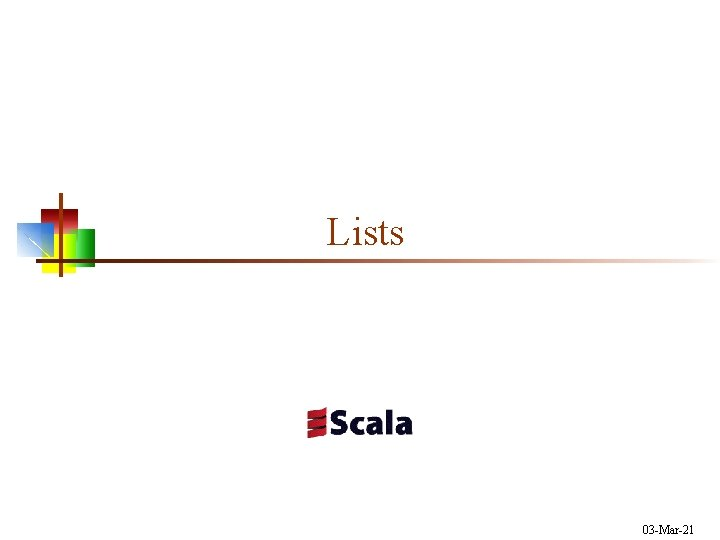Lists 03 -Mar-21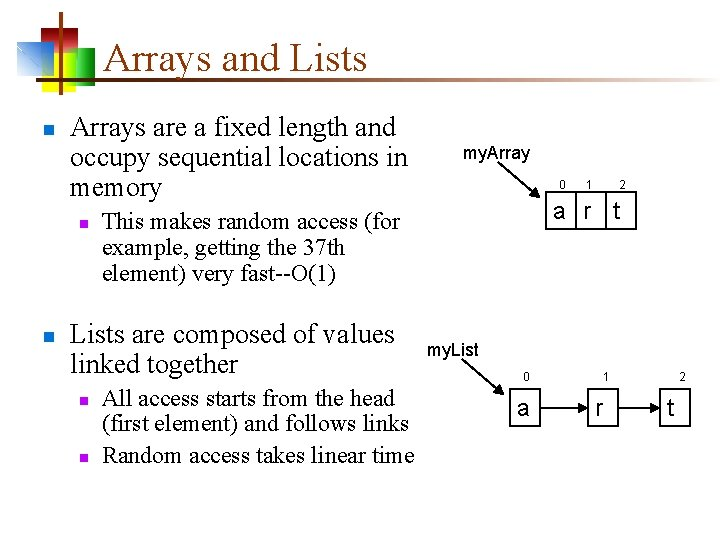Arrays and Lists n Arrays are a fixed length and occupy sequential locations in memory n n n 0 All access starts from the head (first element) and follows links Random access takes linear time 1 2 a r t This makes random access (for example, getting the 37 th element) very fast--O(1) Lists are composed of values linked together n my. Array my. List 0 a 1 r 2 tLists are immutable n n Lists, like Strings, are immutable Because all access is via the head, creating a “new” list is a fast operation my. Longer. List my. List p my. Shorter. List a r t • my. Longer. List looks like List("p", "a", "r", "t"); the "p" is not visible from my. List • my. Shorter. List looks like List("r", "t") • my. List has not been changed--it is immutableList operations n Basic fast (constant time) operations n n n list. head (or list head) returns the first element in the list. tail (or list tail) returns a list with the first element removed value : : list returns a list with value appended to the front list. is. Empty (or list is. Empty ) tests whether the list is empty Some slow (linear time) operations n n n list(i) returns the ith element (starting from 0) of the list. last (or list last) returns the last element in the list. init (or list init) returns a list with the last element removed n n This involves making a complete copy of the list. length (or list length) returns the number of elements in the list. reverse (or list reverse) returns a new list with the elements in reverse order In practice, the slow operations are hardly ever needed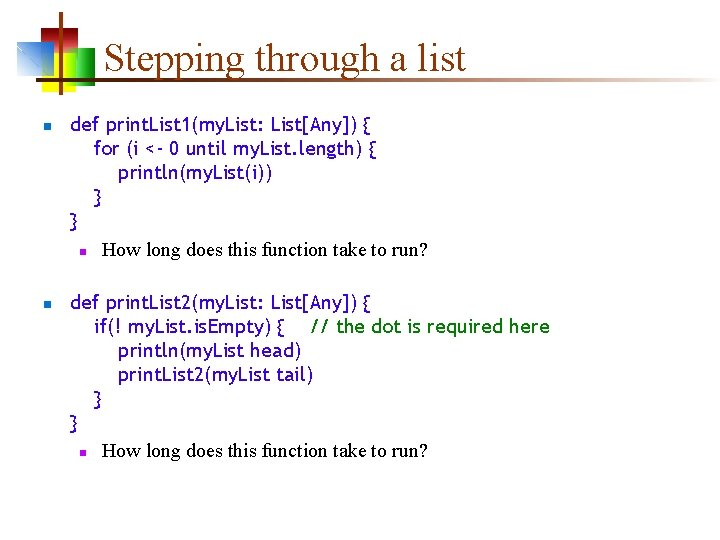Stepping through a list n n def print. List 1(my. List: List[Any]) { for (i <- 0 until my. List. length) { println(my. List(i)) } } n How long does this function take to run? def print. List 2(my. List: List[Any]) { if(! my. List. is. Empty) { // the dot is required here println(my. List head) print. List 2(my. List tail) } } n How long does this function take to run?Basic recursion n n Recursion is when a method calls itself Here’s the basic formula for working with a list: n n if the list is empty return some initial value (often an empty list) else process the head recur with the tail def print. List 2(my. List: List[Any]) { if(! my. List. is. Empty) { println(my. List head) print. List 2(my. List tail) } }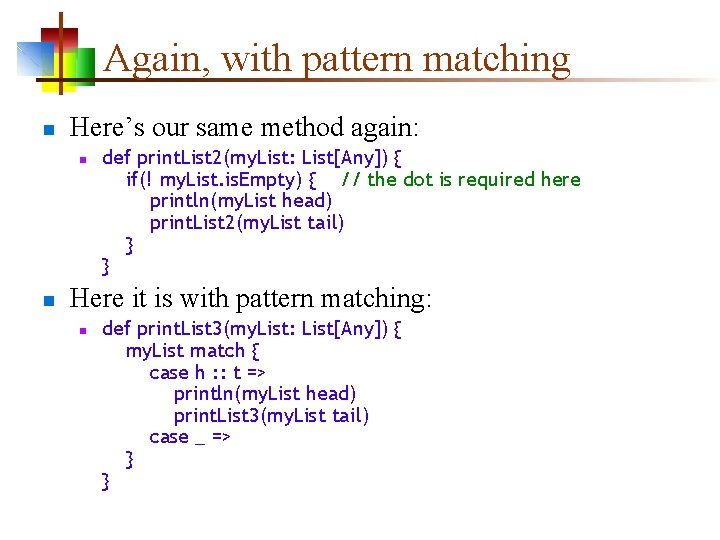Again, with pattern matching n Here’s our same method again: n n def print. List 2(my. List: List[Any]) { if(! my. List. is. Empty) { // the dot is required here println(my. List head) print. List 2(my. List tail) } } Here it is with pattern matching: n def print. List 3(my. List: List[Any]) { my. List match { case h : : t => println(my. List head) print. List 3(my. List tail) case _ => } }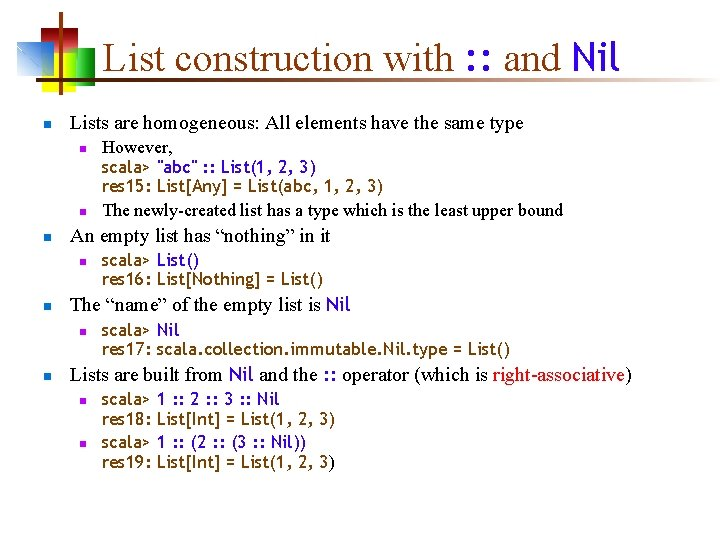List construction with : : and Nil n Lists are homogeneous: All elements have the same type n n n An empty list has “nothing” in it n n scala> List() res 16: List[Nothing] = List() The “name” of the empty list is Nil n n However, scala> "abc" : : List(1, 2, 3) res 15: List[Any] = List(abc, 1, 2, 3) The newly-created list has a type which is the least upper bound scala> Nil res 17: scala. collection. immutable. Nil. type = List() Lists are built from Nil and the : : operator (which is right-associative) n n scala> 1 : : 2 : : 3 : : Nil res 18: List[Int] = List(1, 2, 3) scala> 1 : : (2 : : (3 : : Nil)) res 19: List[Int] = List(1, 2, 3)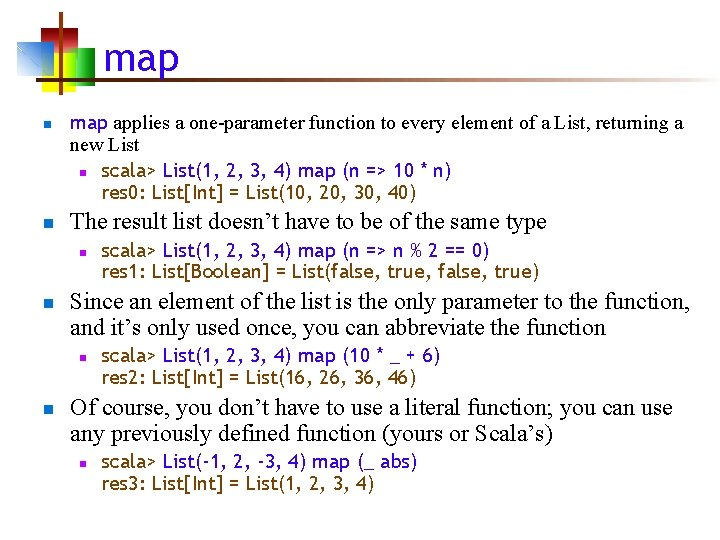map n n map applies a one-parameter function to every element of a List, returning a new List n scala> List(1, 2, 3, 4) map (n => 10 * n) res 0: List[Int] = List(10, 20, 30, 40) The result list doesn’t have to be of the same type n n Since an element of the list is the only parameter to the function, and it’s only used once, you can abbreviate the function n n scala> List(1, 2, 3, 4) map (n => n % 2 == 0) res 1: List[Boolean] = List(false, true, false, true) scala> List(1, 2, 3, 4) map (10 * _ + 6) res 2: List[Int] = List(16, 26, 36, 46) Of course, you don’t have to use a literal function; you can use any previously defined function (yours or Scala’s) n scala> List(-1, 2, -3, 4) map (_ abs) res 3: List[Int] = List(1, 2, 3, 4)flat. Map n flatten “flattens” a list n n flat. Map is like map, but the function given to flat. Map is expected to return a list of values; the resultant list of lists is then “flattened” Syntax: n n n scala> val nested = List(1, 2, 3), List(4, 5)) nested: List[Int]] = List(1, 2, 3), List(4, 5)) scala> nested flatten res 0: List[Int] = List(1, 2, 3, 4, 5) def map[B](f: (A) => B): List[B] def flat. Map[B](f: (A) => Traversable[B]): List[B] Example: n n scala> greeting map (word => word. to. List) res 2: List[Char]] = List(H, e, l, l, o), List(f, r, o, m), List(S, c, a, l, a)) scala> greeting flat. Map (word => word. to. List) res 3: List[Char] = List(H, e, l, l, o, f, r, o, m, S, c, a, l, a)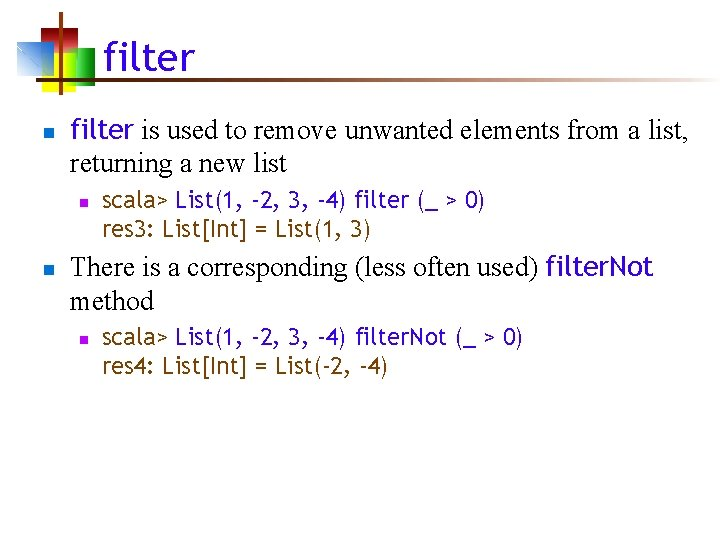filter n filter is used to remove unwanted elements from a list, returning a new list n n scala> List(1, -2, 3, -4) filter (_ > 0) res 3: List[Int] = List(1, 3) There is a corresponding (less often used) filter. Not method n scala> List(1, -2, 3, -4) filter. Not (_ > 0) res 4: List[Int] = List(-2, -4)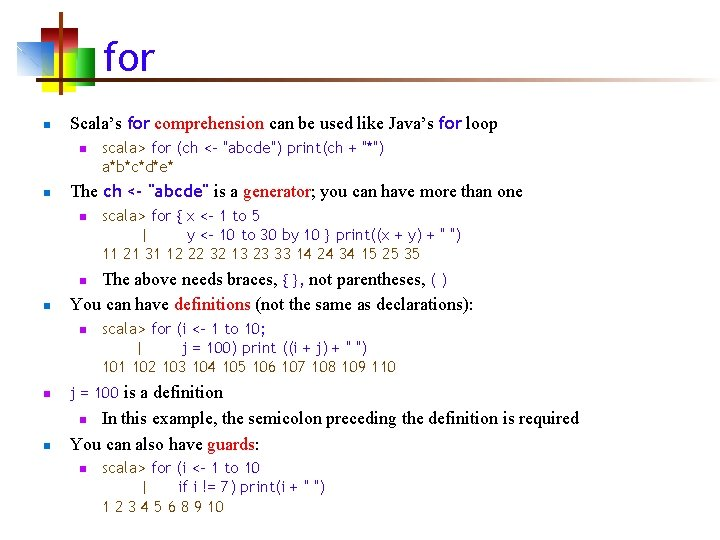for n Scala’s for comprehension can be used like Java’s for loop n n scala> for (ch <- "abcde") print(ch + "*") a*b*c*d*e* The ch <- "abcde" is a generator; you can have more than one n scala> for { x <- 1 to 5 | y <- 10 to 30 by 10 } print((x + y) + " ") 11 21 31 12 22 32 13 23 33 14 24 34 15 25 35 The above needs braces, { }, not parentheses, ( ) You can have definitions (not the same as declarations): n n n scala> for (i <- 1 to 10; | j = 100) print ((i + j) + " ") 101 102 103 104 105 106 107 108 109 110 n j = 100 is a definition n In this example, the semicolon preceding the definition is required You can also have guards: n n scala> for (i <- 1 to 10 | if i != 7) print(i + " ") 1 2 3 4 5 6 8 9 10Another for example n You need to start with a generator, and after that you can have more generators, definitions, and guards n scala> for { i <- 1 to 5 if i % 2 == 0 | k = 100 | j <- 1 to 5 | if j * k < 450 } print((k + 10 * i + j) + " ") 121 122 123 124 141 142 143 144for-yield n n The value of a for comprehension, without a yield, is () With a yield, the value is a list of results (one result for each time through the loop) The syntax is: for (sequence) yield expression Examples: n n scala> for (i <- 1 to 5) yield 10 * i res 12: scala. collection. immutable. Indexed. Seq[Int] = Vector(10, 20, 30, 40, 50) scala> for (n <- List("one", "two", "three")) yield n. substring(0, 2) res 2: List[java. lang. String] = List(on, tw, th)Another for-yield example n Here’s a more complete example (Odersky, p. 125): n n val for. Line. Lengths = for { file <- files. Here // ‘files. Here’ is an array of files if file. get. Name. ends. With(". scala") line <- file. Lines(file) // get an Iterator[String] trimmed = line. trim if trimmed. matches(". *for. *") } yield trimmed. length // get an Array[Int] The above method: n n n n gets each file from an array of files considers only the file with the. scala extension gets an iterator for the lines in the file removes whitespace from the beginning and end of the line looks for “for” within the line (using a regular expression) counts the number of characters in the line returns an array of line lengths of lines containing “for” in scala filesto. List n n n scala> Array(1, 2, 3, 4) to. List res 12: List[Int] = List(1, 2, 3, 4) scala> "abc" to. List res 13: List[Char] = List(a, b, c) scala> Map("apple" -> "red", "banana" -> "yellow") to. List res 14: List[(java. lang. String, java. lang. String)] = List((apple, red), (banana, yellow)) scala> Set("abc", 123) to. List res 16: List[Any] = List(abc, 123) scala> List(1, 2, 3) to. List res 17: List[Int] = List(1, 2, 3)� Also: to. Array, to. String, to. Set, to. MapPattern matching n Given this definition: n n This works: n n n scala> val my. List = List("a", "b", "c") my. List: List[java. lang. String] = List(a, b, c) scala> val List(x, y, z) = my. List x: java. lang. String = a y: java. lang. String = b z: java. lang. String = c But it’s pretty useless unless you know the exact number of items in the list Here’s a better way: n scala> val hd : : tl = my. List hd: java. lang. String = a tl: List[java. lang. String] = List(b, c)Example program object English. To. German { def main(args: Array[String]) { println(translate("Scala is a wonderful language !")) } def translate(english: String) = { val dictionary = Map("a" -> "ein", "is" -> "ist", "language" -> "Sprache", "wonderful" -> "wunderbar") def lookup(word: String) = { if (dictionary contains word) dictionary(word) else word } (english. split(" ") map (lookup(_))). mk. String(" ") } } Output: Scala ist ein wunderbar Sprache !The End